•••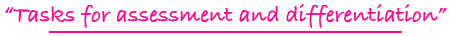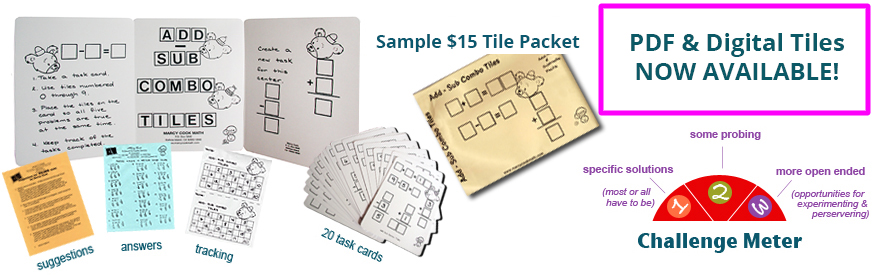Tiling Task Card Packets include 20 unique task cards on card stock, 3 backdrop cards, tracking sheets, suggestions and answer sheets.  These are contained in a printed manila envelope for convenient storing.  A set of number tiles 0 - 9 is  needed for each task card.

4 - 7

### COMPARE A TILE

comparing common fractions with like and unlike denominators; dealing with inequalities ( )

Challenge Level: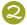\$15.00

5 - 8

### CONVERT A TILE

changing common fractions to decimals and decimals to common fractions

Challenge Level:AVAILABLE IN PDF FORMAT ONLY
(You will receive both color and black and white files for printing.)\$10.00

5 - 8

### DECIMAL PLACE VALUE TILES I

forming 4 specified numbers with tenths and hundredths places involved

Challenge Level:\$15.00

5 - 8

### DECIMAL PLACE VALUE TILES II

experimenting with digits to form numbers with 1,  2, or 3 digits to the right of the decimal point

Challenge Level:\$15.00

4 - 8

### DECIMAL ROUND THE NUMBER TILES

rounding numbers to the nearest tenths, hundredths, or thousandths

Challenge Level:AVAILABLE IN PDF FORMAT ONLY
(You will receive both color and black and white files for printing.)\$10.00

5 - 8

### DIVI-DECI TILES

dividing decimal fractions

Challenge Level:\$15.00

4 - 7

### EQUI-TILES

forming equivalent common fractions

Challenge Level:\$15.00

4 - 7

### FRACT-ADD TILES

adding common fractions with like and unlike denominators

Challenge Level:\$15.00

4 - 7

### FRACT-SUB TILES

subtracting common fractions with like and unlike denominators

Challenge Level:\$15.00

4 - 7

### FRACTION & NUMBER LINE TILES

comparing common fractions ( )using a visual model of a number line between zero and one

Challenge Level:AVAILABLE IN PDF FORMAT ONLY
(You will receive both color and black and white files for printing.)\$10.00

4 - 7

### FRACTION COMPARISON TILES

comparing two fractions with different numerators and different denominators using visual models to record comparisons with symbols

Challenge Level:\$15.00

4 - 8

### FRACTION TILES

experimenting with number tiles to form common fractions; example: fractions equivalent to 3/4

Challenge Level: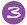\$15.00

4 - 8

### FRACTIONAL PART TILES

finding fractional parts of whole numbers; examples: 1/3 of 39; less than 1/4 of 100

Challenge Level:\$15.00

5 - 8

### MULTI-DECI TILES

multiplying decimal fractions

Challenge Level:\$15.00

5 - 8

### PERCENTAGE TILES I

changing common fractions to decimals and decimals to percents and vice versa

Challenge Level: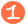\$15.00

5 - 8

### PERCENTAGE TILES II

computing the three basic types of percent problems

Challenge Level:\$15.00

3 - 6

### PICTURE FRACTION TILES

forming common fractions from pictures, including equivalent fractions and geometry vocabulary for part of a total group

Challenge Level:\$15.00

5 - 8

### +/- DECI TILES

addition and subtraction of decimal fractions

Challenge Level:\$15.00

3 - 6

### VIEW A FRACTION TILES

forming common fractions from pictures, including equivalent fractions and comparisons of fractions

Challenge Level:\$15.00

5 - 8

### VISUALIZING TILES

dealing with representational models for common fractions, percent, ratio, and square numbers

Challenge Level:\$15.00